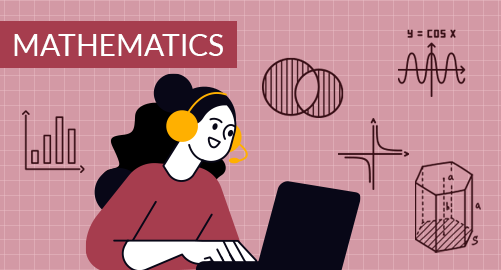#### Need Help?

Get in touch with usMathematics

Grade 9 Mathematics covers Essentials of Geometry, Reasoning and Proof, Congruent Triangles, Similarity, Right Triangles and Trigonometry, Quadrilaterals and Circles, Measuring Length, Area, Surface area, Volume

## What you'll learn

• 1

Essentials of Geometry: Identify Points, Lines, and Planes, Use Segments and Congruence, Use Midpoint and Distance Formulas, Measure and Classify Angles, Describe Angle Pair Relationships, Classify Polygons, Find Perimeter, Circumference, and Area.

• 2

Reasoning and Proof: Use Inductive Reasoning, Analyze Conditional Statements, Apply Deductive Reasoning, Use Postulates and Diagrams, Reason Using Properties from Algebra, Prove Statements about Segments and Angles, Prove Angle Pair Relationships.

• 3

Congruent Triangles: Apply Triangle Sum Properties, Apply Congruence and Triangles, Prove Triangles Congruent by SSS, SAS, HL, ASA and AAS, Use Congruent Triangles, Use Isosceles and Equilateral Triangles, Perform Congruence Transformations, Midsegment Theorem, Coordinate Proof, Perpendicular Bisectors, Angle Bisectors of Triangles, Use Medians and Altitudes, Inequalities in a Triangle, Inequalities in Two Triangles and Indirect Proof.

• 4

Similarity: Ratios, Proportions, and the Geometric Mean, Use Proportions to Solve Geometry Problems, Prove Triangles Similar by AA, Use Proportionality Theorems, Perform Similarity Transformations.

• 5

Right Triangles and Trigonometry: Apply the Pythagorean Theorem, Use the Converse of the Pythagorean Theorem, Use Similar Right Triangles, Special Right Triangles, Apply the Sine and Cosine Ratios, Solve Right Triangles.

• 6

Quadrilaterals and Circles: Find Angle Measures in Polygons, Properties of Parallelograms, Properties of Rectangles, Rhombuses, Trapezoids and Kites, Squares, Use Properties of Tangents, Find Arc Measures, Properties of Chords, Inscribed Angles and Polygons, Other Angle Relationships in Circles, Segment Lengths in Circles, Write and Graph Equations of Circles.

• 7

Measuring Length, Area, Surface area, Volume: Areas of Triangles, Parallelograms, Trapezoids, Rhombuses, and Kites, Perimeter and Area of Similar Figures, Circumference and Arc Length, Areas of Circles, Regular Polygons, Use Geometric Probability, Explore Solids, Surface Area of Prisms, Cylinders, Pyramids and Cones, Volume of Prisms and Cylinders, Pyramids, Cones, Explore Similar Solids.

## Skills you'll learnIdentify an appropriate mathematical rule or procedure based on the classification of a given expressionExplain how an approximated value relates to the actual valueIdentify mathematical information from graphical, numerical, analytical, and/or verbal representationsDescribe the relationships among different. representations of functions and their derivativesExplain the meaning of mathematical solutions in contextIdentify an appropriate mathematical definition, theorem, or test to applyUse precise mathematical language and appropriate units of measure and symbols